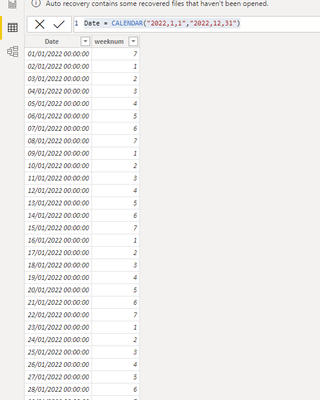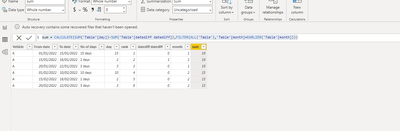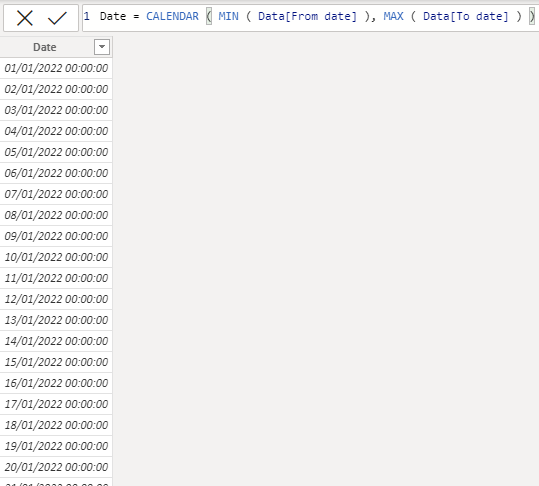cancel
Showing results for
Did you mean:Helper IV

## calculate number of days

hi

how can i write a dax formula to calculate the number of working days ( only count the date, not time) in each month as below.

Number of working days in jan should be 19days ( 15+2+3 =20 but minus 1 as there is overlap in day 15/1/2022).

Number of working days in feb should be 15 days

 Vehicle From date To date No of days A 1/1/2022 15/1/2022 15 days A 15/1/2022 16/1/2022 2 days A 20/1/2022 22/1/2022 3 days A 1/2/2022 10/2/2022 10 days A 15/2/2022 16/2/2022 2 days A 20/2/2022 22/2/2022 3 days

thanks

1 ACCEPTED SOLUTIONCommunity Support

HI @ktt777 ,

Based on the data you provided, try the following steps.

Step1,create a date table:

``Date = CALENDAR("2022,1,1","2022,12,31")``Step2, calculate date between two date:

``day = CALCULATE(COUNTROWS('Date'),FILTER('Date','Date'[Date]>='Table'[From date]&&'Date'[Date]<='Table'[To date]))``

Then use the below dax to create new column in order to calculate overlap  day：

``rank = RANKX('Table','Table'[From date],,ASC,Dense)``
``````datediff datediff =
IF (
'Table'[rank] <> 1,
IF (
DATEDIFF (
'Table'[From date],
CALCULATE (
MAX ( 'Table'[To date] ),
FILTER ( ALL ( 'Table' ), 'Table'[rank] = EARLIER ( 'Table'[rank] ) - 1 )
),
DAY
) < 0,
0,
DATEDIFF (
'Table'[From date],
CALCULATE (
MAX ( 'Table'[To date] ),
FILTER ( ALL ( 'Table' ), 'Table'[rank] = EARLIER ( 'Table'[rank] ) - 1 )
),
DAY
) + 1
),
0
)
``````

Final to get sum  value for every month:

``month = MONTH('Table'[From date])``

``sum = CALCULATE(SUM('Table'[day])-SUM('Table'[datediff datediff]),FILTER(ALL('Table'),'Table'[month]=EARLIER('Table'[month])))``

Output:Best Regards

Lucien

3 REPLIES 3Community Support

HI @ktt777 ,

Based on the data you provided, try the following steps.

Step1,create a date table:

``Date = CALENDAR("2022,1,1","2022,12,31")``Step2, calculate date between two date:

``day = CALCULATE(COUNTROWS('Date'),FILTER('Date','Date'[Date]>='Table'[From date]&&'Date'[Date]<='Table'[To date]))``

Then use the below dax to create new column in order to calculate overlap  day：

``rank = RANKX('Table','Table'[From date],,ASC,Dense)``
``````datediff datediff =
IF (
'Table'[rank] <> 1,
IF (
DATEDIFF (
'Table'[From date],
CALCULATE (
MAX ( 'Table'[To date] ),
FILTER ( ALL ( 'Table' ), 'Table'[rank] = EARLIER ( 'Table'[rank] ) - 1 )
),
DAY
) < 0,
0,
DATEDIFF (
'Table'[From date],
CALCULATE (
MAX ( 'Table'[To date] ),
FILTER ( ALL ( 'Table' ), 'Table'[rank] = EARLIER ( 'Table'[rank] ) - 1 )
),
DAY
) + 1
),
0
)
``````

Final to get sum  value for every month:

``month = MONTH('Table'[From date])``

``sum = CALCULATE(SUM('Table'[day])-SUM('Table'[datediff datediff]),FILTER(ALL('Table'),'Table'[month]=EARLIER('Table'[month])))``

Output:Best Regards

LucienSuper User

Hi @ktt777

Sample file https://we.tl/t-IuoukK27zQ
Here is a measure to calculate monthly working days. However, removing the overlapping days shall be a bet complex as there is no consistant criteria. If this is a msut do then I need to write more DAX.``````Number of Days =
SUMX (
VALUES ( 'Date'[Date].[Month] ),
CALCULATE (
SUMX (
CROSSJOIN ( Data, VALUES ('Date'[Date] ) ),
VAR CurrentDate = 'Date'[Date]
VAR FromDate = Data[From date]
VAR ToDate = Data[To date]
RETURN
IF ( CurrentDate >= FromDate && CurrentDate <= ToDate, 1 )
)
)
)``````Super User

@ktt777 , Create a new column like

COUNTROWS(FILTER(ADDCOLUMNS(CALENDAR(Table[from Date],Table[to Date]),"WorkDay", if(WEEKDAY([Date],2) <6,1,0)),[WorkDay] =1))

How to calculate Business Days/ Workdays, with or without date table: https://youtu.be/Qv4wT8_P-AA